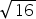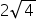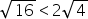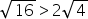Mathematics
Easy

Question

# Compareto.

## EquivalentNot equivalentHint:

## The correct answer is: Equivalent

### We have to compare √16 to 2√4.We have, √16 = 4.And, 2√4 = 2×2 = 4.So, √16 = 2√4.Hence, the correct option is A.

The root of a perfect square number is always an integer.

### Related Questions to study#### With Turito Foundation.#### Get an Expert Advice From Turito.